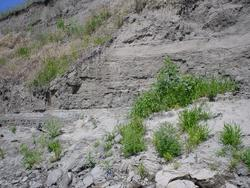# Three-Point Problem by Simultaneous Linear Equations

## SummaryStudents are introduced to the use of linear algebra in an intuitive and accessible way, through classroom activity and homework set. The familiar three-point problem is cast in terms of three dimensional analytic geometry, fostering understanding of mathematical models for simple geometric forms. The suitability of a planar representation of geological features such as sedimentary horizons or fault surfaces is discussed in class. Preparatory to developing a useful algorithm, a system of equations is developed, put into matrix form (which most have seen before, but never applied), and solved for slopes and intercept. Finally, the slopes are resolved into geologically familiar strike and dip form.

Used this activity? Share your experiences and modifications

## Learning Goals

Those not familiar with the 3-point problem will learn how to determine the attitude of a (planar) bed from given elevations at three locations.

All students will learn:

• to use simultaneous linear equations to provide a quick and accurate solution of the 3-Point problem
• to use mathematical models to approximate realistic geologic phenomena
• the value of a Linear Algebraic approach to solving Systems of Linear Equations

The exercise can also serve as an intuitive springboard for subsequent learning in data fitting, parameter estimation, experiment design, data resolution, and factor analysis.

## Context for Use

The activity can be used in courses on structural geology, field methods, and stratigraphy where determining the attitude of planar features is important, or courses on quantitative methods in the geosciences as an introduction to linear algebra. Computational tools involved can be either the Excel spreadsheet, a downloadable linear-equation solver called LINEQ, or the basic MatLab package. An Excel simulator is provided to generate sample problems.

The concepts of simultaneous linear equations and matrix representation are assumed, though it has been found useful to review them, along with matrix multiplication and inverses, as part of the lesson. It is helpful, though not required, for the students to have a visual understanding of the 3-point problem in advance.

## Description and Teaching Materials

Notes about the Analytic Geometry (Acrobat (PDF) 154kB Aug5 04) involved in this problem.

Versions of the exercise for different calculation methods:

Useful Tools:

Examples:

Preparatory material: A prior assignment on Analytic Geometry (Acrobat (PDF) 316kB Jun30 04)

## Teaching Notes and Tips

General teaching notes (Acrobat (PDF) 4kB Jun30 04)

This approach to the 3-point problem has been presented several times to students who have already seen more traditional techniques of solution; they have generally accepted it as more convenient and useful than their previous experience. Frankly, I believe that their impressions rest largely on their already having the geometry well in mind!

Though most students these days have previously had glimpses of matrix solutions to linear equations, they often need a refresher. I have found it easy to do in classroom discussion, reminding them of the rules of matrix multiplication and pointing out the parallel to the linear equations that we've just written for depths to a plane at three different locations.

A major benefit of this approach has been that the concepts of simultaneous linear equations and linear algebra (i.e., matrix methods) can be recognized as the easy way to do a problem which students already understand. The practical problem brings their prior knowledge to the fore, and helps them seek ways to apply those skills elsewhere.

Productive directions for extension include:

• factor analysis of geochemical data (see example exam problem)
• least squares fitting through development of the overdetermined problem as u = [ATA]-1 AT z
• discussion of data resolution and experiment design by considering the placement of the drillholes in the problem

Those using the Excel version may find it handy to refer students to the Excel Help for Students

## Assessment

Progress may be effectively assessed by devising exam problems (e.g., using the simulator) requiring use of the tools and techniques developed.

## References and Resources

Alternate approaches to the 3-Point problem may be found in most structural geology textbooks.

Vacher, H.L. (2000). Computational Geology 12: Cramer's rule and the three-point problem. J. Geosci. Ed., 48(4), 522-532.
Dr. Vacher provides a solution to this problem using linear algebra.